# Pulsar sounds: Sonification of a pulsar (Vela, PSR B0833-45)

Written by Paul Bourke
October 2005

Pulsars are neutron stars with the mass of a star compressed to perhaps 20km diameter. Pulses of radiation are emitted along the poles of an immensely strong magnetic field, we can measure that beam of radiation if it happens to cross the Earths path. The pulsar being used here as an example is in the Vela supernova remnant, sometimes simply called the Vela pulsar but more precisely known as PSR B0833-45. The purpose of this exercise was to investigate how such events could be sonified.

Original pulses

This pulsar is rotating at close to 11 fps, this is the rate at which we detect a pulse from one of the poles arriving at Earth. The raw data sampled at 30KHz is shown below, this can be listened to directly since it naturally has energy in the audio band. In what follows a very small section of the time series is shown along with a audio movie of the entire recorded sequence, this format will be used for the rest of this page.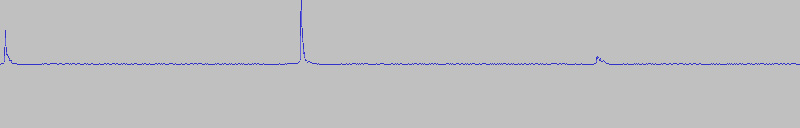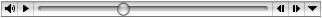1KHz carrier

In order to raise the frequency the envelop of the pulsar pulse can be used to modulate a sinusoid, otherwise known as a carrier in AM radio (amplitude modulation). This carrier can be any frequency up to the Nyquist criteria of the sampling frequency. The sample below is a 1000Hz carrier, an interesting variant for wider pulses is to vary the frequency of the carrier based upon the pulse peak height, this is otherwise known as FM (frequency modulation).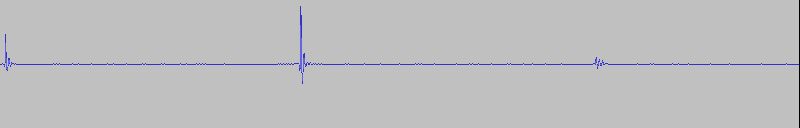Modulated white noise

The envelop can be used to modulate white noise, Gaussian distributed noise, or some other noise distribution.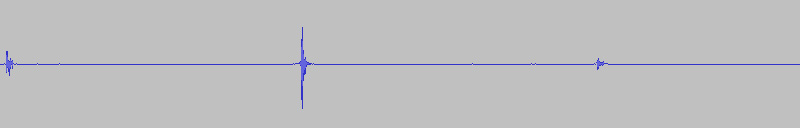Modulated square wave

....or a square wave.Triggered options

There is clearly a limit to the variation that can be achieved when using the pulsar pulse envelop to modulate another series especially given the short time period of the pulses. Perhaps a more interesting option is to use the pulse peak to trigger an "instrument". In this example a sinusoidal envelop is triggered where the frequency of the signal is proportional to the height of the pulse peak. High pulse peak results in a high frequency burst, a low pulse height results in a low frequency burst. The range of frequencies spanned the human audio range. The result is a whistling effect.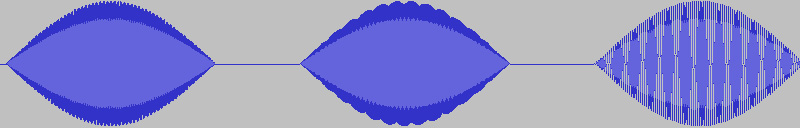The following is similar except now the resulting burst is a exponentially decaying sinusoid. This gives a sharp popping sound.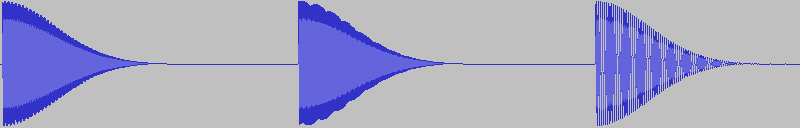Equal tempered scale, 3 octaves

Instead of using a continuous range of frequencies, one can also use an equal tempered scale, something we are more used to with classical keyboard instruments. The allowed frequencies are in precise ratios, the example here uses the 12 notes of the chromatic scale, the following example spans 3 octaves from A4 (440Hz). In this example the amplitude of the burst is also proportional to the pulsar pulse height.